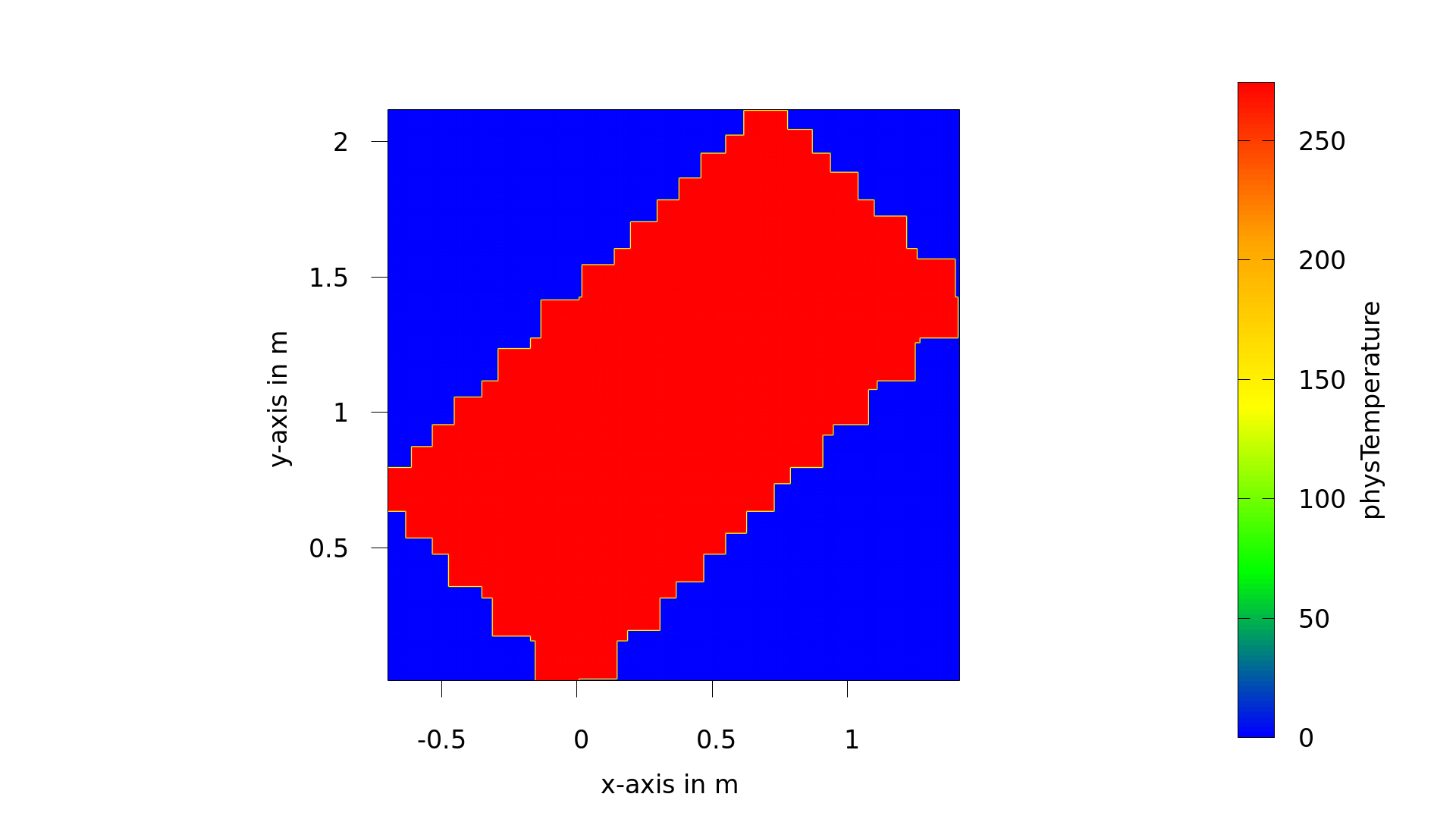OpenLB – Open Source Lattice Boltzmann Code Forums on OpenLB General Topics How to create the cuboidGeometry for a inclined 2D cuboid geometry

Viewing 5 posts - 1 through 5 (of 5 total)
• Author
Posts
• #6126
Fany
Participant

Hi everyone,
I am trying to simulate a inclined 2D cuboid geometry. But the computational domain was below (rotating the geometry of rayleibenrd2d example). Whether did that have a problem in the functor CuboidGeometry?#6127
Gloriousface
Participant

Hi Fany:
You can use BlockGeometryXD to specify the name of the cell with its location. Firstly you instantiate one object with BlockGeometryXD, BlockGeometryXD<T> &BG=superGeometry.getExtendedBlockGeometry(iC), where iC is the index of the block. Then the size of this BG is get with Nx=BG.getNx() and Ny=BG.getNy(); Then in a for loop with ix from 2 to Nx-2, iy from 2 to Ny-2, get the physical position of the cell with BG.getPhysR(phr,ix,iy); if the physical position statisfies the requiement, rename the cell with BG.get(ix,iy)=2 for example.

#6128
Gloriousface
Participant

one example snippet to wrok is like the following:
for(int iC=0;iC<4;++iC){
BlockGeometry2D<T>& BGeo=superGeometry.getExtendedBlockGeometry(iC);
int Nx=BGeo.getNx();
int Ny=BGeo.getNy();
for(int ix=2;ix<=Nx-2;++ix){
for(int iy=2;iy<Ny-2;++iy){
BGeo.getPhysR(phR,ix,iy);
indx=phR;
indy=phR;
if(indx>10 && indx<15 && indy>20 && indy<50){
BGeo.get(ix,iy)=2;
}
}
}

clout<<“BlockGeometry “<<iC<<” prepared!”<<endl;
}

#6129
Fany
Participant

Dear Gloriousface,
Thanks very much for your suggestion of creating the geometry boundary. Is that used to rename the boundary but not using the class supergeometry.rename in OpenLB?
Actually, I feel the serrated boundary was caused by the class CuboidGeometry2D because the boundary would changed with the different parameter noofCuboids and increasingly approximate to a rectangle with the increasing noofcuboids. I mean the problem may occurred in the process of constructing cuboid, shrinking remaining cuboids, distributing the load balancer and renaming material. I adapted the functor IndicatorCuboid2D to creating the 2D cuboid with a rotation angle. But it is normal when the angle=90, but abnormal when the angle was 45 (seen in the above image).

`/// === 2nd Step: Prepare Geometry ===
std::vector<T> extend(2,T());
extend = lx;
extend = ly;
std::vector<T> origin(2,T());
IndicatorCuboid2D<T> cuboid(extend, origin, theta);

/// Instantiation of a cuboidGeometry with weights
#ifdef PARALLEL_MODE_MPI
const int noOfCuboids = 16*singleton::mpi().getSize();
#else
const int noOfCuboids = 7;
#endif
CuboidGeometry2D<T> cuboidGeometry(cuboid, converter.getPhysDeltaX(), noOfCuboids);

// cuboidGeometry.setPeriodicity(true, false);

prepareGeometry(superGeometry, converter);

#6130
Fany
Participant

Hi Gloriousface,
Here is the code of renaming the material numbers.
`
OstreamManager clout(std::cout,”prepareGeometry”);
clout << “Prepare Geometry …” << std::endl;

superGeometry.rename(0,2);
superGeometry.rename(2,1,0,1);

std::vector<T> extend( 2, T(0) );
extend = lx;
extend = converter.getPhysLength(1);
std::vector<T> origin( 2, T(0) );
IndicatorCuboid2D<T> bottom(extend, origin, theta);

origin = ly-converter.getPhysLength(1);
IndicatorCuboid2D<T> top(extend, origin, theta);

origin = lx/2.;
origin = converter.getPhysLength(1);
extend = converter.getPhysLength(1);
extend = converter.getPhysLength(1);
IndicatorCuboid2D<T> perturbation(extend, origin, theta);

/// Set material numbers for bottom, top and pertubation
superGeometry.rename(2,2,1,bottom);
superGeometry.rename(2,3,1,top);
superGeometry.rename(1,4,perturbation);

/// Removes all not needed boundary voxels outside the surface
superGeometry.clean();
/// Removes all not needed boundary voxels inside the surface
superGeometry.innerClean();
superGeometry.checkForErrors();

superGeometry.print();

clout << “Prepare Geometry … OK” << std::endl;

Viewing 5 posts - 1 through 5 (of 5 total)
• You must be logged in to reply to this topic.